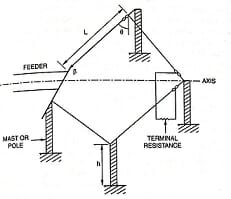# Rhombic Antenna | features & advantages## Rhombic Antenna

The rhombic antenna is based on the principle of travelling wave radiator. By application of return conductor, two wires are pulled apart at a point so that diamond or rhombus shape is formed as shown in the figure below. If the two wires (i.e., two sides of rhombus) are pulled apart to such an extent that the four lobes are combined together, then the additional gain is achieved. Also, there is no problem with proper termination to avoid the reflection. This is the basis of the rhombic antenna.

This is an antenna that is in the shape of a rhombus. It is usually terminated in a resistance. The side of the rhombus, the angle between the sides, the elevation, termination, and height above the earth are chosen to obtain the desired radiation characteristics. A typical Rhombic antenna and radiation pattern are shown in the figure below.

## Salient features of Rhombic antenna

1. It is a long wire antenna and consists of four non-resonant wires.
2. It provides greater directivity than the V antenna.
3. Its bandwidth is high.
4. It is an HF non-resonant antenna.
5. This is very useful for point-to-point communications.
6. It is a traveling wave antenna and there are no reflections.
7. It also finds wide applications where the angle of elevation of the main lobe (measured from the plane of the antenna to the radiation axis) is less than 30°.
8. At an elevation angle above 30°, the gain is very low for practical applications.
9. The length of equal radiators varies from 2 to 8 λ.
10. The tilt angle, ϕ varies between 40° and 75°.
11. ϕ is determined from leg length.
12. The terminating resistance is about 800 Ω.
13. The input impedance of the Rhombic antenna lies between 650 to 700Ω.
14. The directivity of the Rhombic antenna varies between 20 and 90.
15. The power gain lies between 15 and 60 after taking power loss in terminating resistance into account.
16. It is a very useful antenna for transmission and reception in the HF band.
17. It is easy and cheap to erect.
18. The directivity of each wire is $D\left ( \theta \right )=\frac{60I}{r}sin\theta \left [ \frac{sin\left [ \frac{\pi l}{\lambda }\left ( 1-cos\theta \right ) \right ]}{\left ( 1-cos\theta \right )} \right ]$

Where

I = the magnitude of the current in element

θ = the polar angle

λ = wavelength

r = the distance from the radiator to the elevation point.

## Design parameters & equations

The design parameters of the Rhombic antenna are:

1. Rhombic height, H
2. The angle of elevation, ϕ
3. Wire length, I.

The design equations are

$H=\frac{\lambda }{4sin \Delta}$

Δ = elevation angle

Δ is complement of tilt angle, ϕ

(sin ϕ = cos Δ)

$l=\frac{\lambda }{2 cos ^{2}\phi }=\frac{\lambda }{2 sin ^{2}\Delta }$

1. An end-to-end receiving array of a number of rhombics can be designed to form a Multiple Unit Steerable Antenna (MUSA) system which constitutes the present-day ultimate for long-distance short wave reception of horizontally polarized down coming waves.
2. The greatest advantage of the rhombic antenna is that the input impedance and radiation pattern do not change rapidly over a considerable frequency range as compared to any other system say of resonant dipoles.
3. Rhombic antenna is a highly directional broad-band antenna with the greatest radiated or received power along the main axis or longer diagonal.
4. It is a very efficient and widely employed antenna for radio communication where enough space necessary for its installation is no problem.
5. It is simple and cheap to erect.
6. Input impedance is twice to that obtainable from a single side radiator.
7. Short wave antennas of this kind required only a low height.
8. Rhombic antenna is un-tuned and is a useful wideband antenna suitable for rapid switching from one working frequency to another frequency.